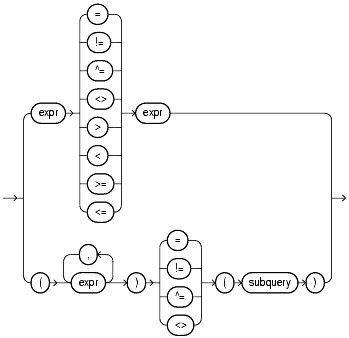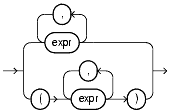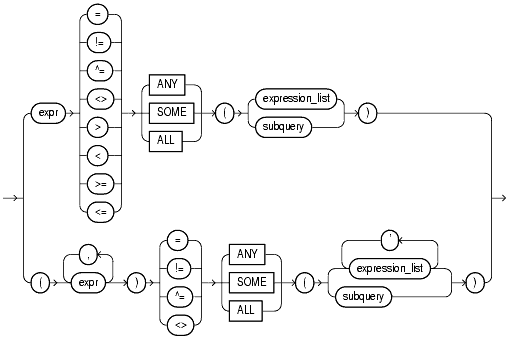## Comparison Conditions

Comparison conditions compare one expression with another. The result of such a comparison can be `TRUE`, `FALSE`, or `UNKNOWN`.

 Note: Large objects (LOBs) are not supported in comparison conditions. However, you can use PL/SQL programs for comparisons on `CLOB` data.

Table 5-2 lists comparison conditions.

##### Table 5-2   Comparison Conditions
Type of Condition Purpose Example
```=
```

Equality test.

```SELECT *
FROM employees
WHERE salary = 2500;
```
```!=
^=
<   >
¬=
```

Inequality test. Some forms of the inequality condition may be unavailable on some platforms.

```SELECT *
FROM employees
WHERE salary != 2500;
```
```>

<
```

"Greater than" and "less than" tests.

```SELECT * FROM employees
WHERE salary > 2500;
SELECT * FROM employees
WHERE salary < 2500;
```
```>=

<=
```

"Greater than or equal to" and "less than or equal to" tests.

```SELECT * FROM employees
WHERE salary >= 2500;
SELECT * FROM employees
WHERE salary <= 2500;
```
```ANY
SOME
```

Compares a value to each value in a list or returned by a query. Must be preceded by =, !=, >, <, <=, >=.

Evaluates to `FALSE` if the query returns no rows.

```SELECT * FROM employees
WHERE salary = ANY
(SELECT salary
FROM employees
WHERE department_id = 30);
```
```ALL
```

Compares a value to every value in a list or returned by a query. Must be preceded by =, !=, >, <, <=, >=.

Evaluates to `TRUE` if the query returns no rows.

```SELECT * FROM employees
WHERE salary >=
ALL ( 1400, 3000);
```

### Simple Comparison Conditions

A simple comparison condition specifies a comparison with expressions or subquery results.

simple_comparison_condition::=Text description of simple_comparison_condition

expression_list::=Text description of expression_list

If you use the lower form of this condition (with multiple expressions to the left of the operator), then you must use the lower form of the `expression_list`, and the values returned by the subquery must match in number and datatype the expressions in `expression_list`.

### Group Comparison Conditions

A group comparison condition specifies a comparison with any or all members in a list or subquery.

group_comparison_condition::=Text description of group_comparison_condition

expression_list::=Text description of expression_list

If you use the upper form of this condition (with a single expression to the left of the operator), then you must use the upper form of `expression_list`. If you use the lower form of this condition (with multiple expressions to the left of the operator), then you must use the lower form of `expression_list`, and the expressions in each `expression_list` must match in number and datatype the expressions to the left of the operator.